# for the Calculation of Algebraic Surfaces

## Richard Morris

### Department of Statistics, University of Leeds

A Client-Server System for the Visualisation of Algebraic Surfaces on the Web full paper (PDF).

# Slides# Abstract

Algebraic surfaces, defined as the zero set of a polynomial function in three variables, present particular problem for visualizing, especially if the surface contains singularities. Most algorithms for constructing a polygonization of the surface will miss the singular points. We present an algorithm for polygonizing such surfaces which attempts to get accurate representations of the singular points. A client-server approach is used to enable the visualization of the polygonal mesh in a web browser. The client is a Java applet which uses the Javaview library which allows the 3D rotation and scaling of the object. The server is a C program which takes the definition of the surface and calculates the polygonization. The client connect to the server using the CGI protocol. This system allows algebraic surfaces to be viewed in any web browser on any platform.

# Key Features

• Calculates 3D models of Algebraic Surfaces
i.e. solutions of f(x,y,z) = 0
• Special attention is paid to surfaces containing singularities
• Uses Java/Javaview which allows it to run on any platform
• Javaview provides interactive rotation of 3D models
• Client-Server approach allows legacy code to be used and minimises download time

# A Brief Tour of Algebraic Surfaces

Many famous surfaces are defined as algebraic surfaces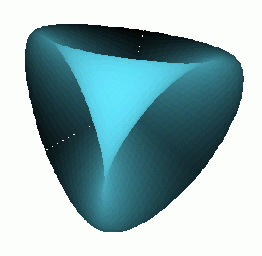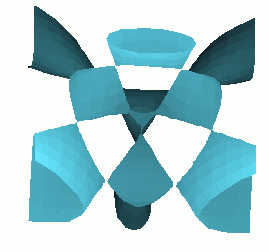Steiner's Roman Surface Kummer Surface Boys Surface

The Kummer surfaces form a parametrised family of surface.

# Singular Surface

Of particular interest are surfaces which contain singularities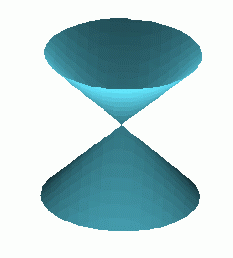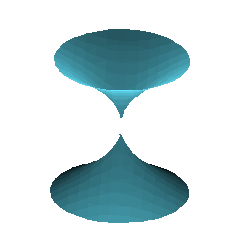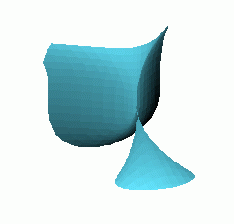A1: x^2 + y^2 - z^2=0 A3: x^2 + y^2 - z^4=0 D4: x^2 y - y^3 - z^2=0 E7: x^3-x y^3 - z^2 =0

These provide a particular challenge for accurate rendering.

# Other surfaces can be very degenerate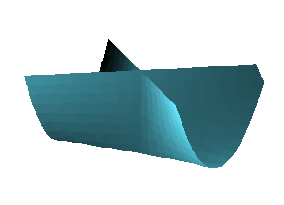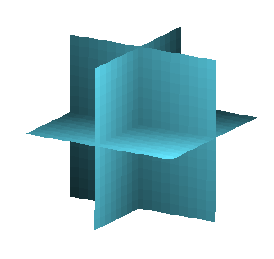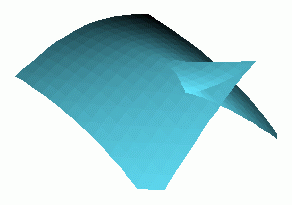Cross Cap: x^2 y - z^2 = 0 triple point: x y z = 0 Swallowtail: 4 z^3 y^2 - 27 y^4 + 16 x z^4 -128 x^2 z^2 - 144 x y^2 z + 256 x^3 = 0

The swallowtail surface is the discriminate surface for the set of quartic polynomials x^4 + a x^2 + b x + c. Giving values of a,b,c where the polynomial has a repeated root.

# The User Interface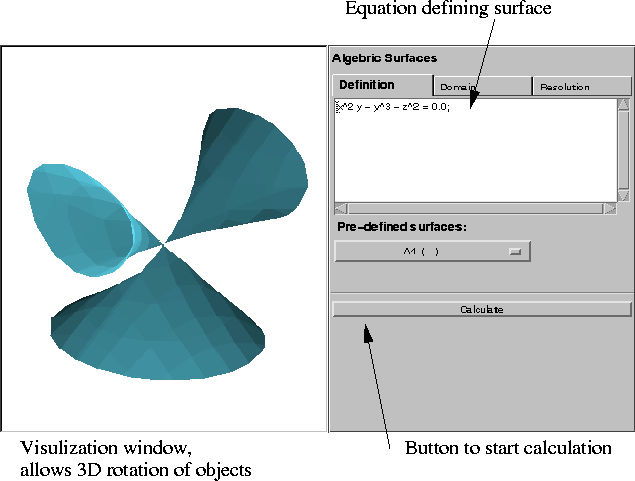Fairly sophisticated syntax allows substitutions, vector operations and symbolic differentiation.

# Overview of operation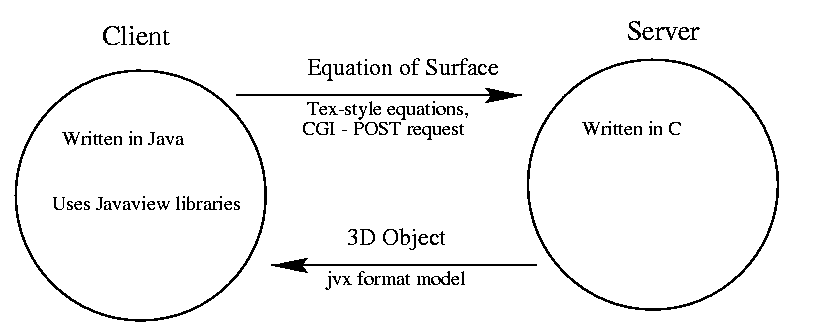# Calculation of Surface

• Uses Bernstein basis to represent polynomials
• Uses oct-tree recursive sub division
• Easy test for possible existence of zeros allows early pruning of the search tree
Algorithm adapted from a 2D algorithm for Algebraic Curves written by A Geisow.

# Bernstein Polynomials

In 1-D a polynomial B(x) of degree n can be written as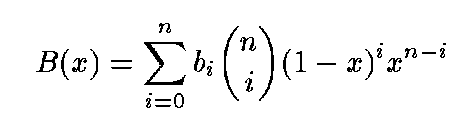where the bi are the Bernstein coefficients. Easy extension to 3-D.

## Test for zeros

If bi > 0 for i= 0 ... n

Then B(x) >0 for x in [0,1]

This gives a quick test to see whether a region contains a zero. The reverse does not necessarily follow.

# Testing for singularities

f(x,y,z) will have a singularity at (x,y,z) if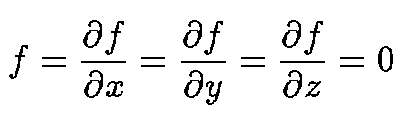This may be an isolated point, a double cone or some more complicated singularity depending on the values of the higher derivatives.

A test on Bernstein coefficients is used to test for the possible presence of singularities

# Basic operation of algorithm

2. Subdivide into 8 smaller boxes
3. use test for zeros to eliminate boxes which do not contain a part of the surface
4. repeat stages 2,3,4 until fixed depth reached
5. Find solutions on the edges, faces and interior of smaller box
6. Link singularities together to create a polygonization of the surface

# Finding Solutions

In each smaller box various different types of solution are found.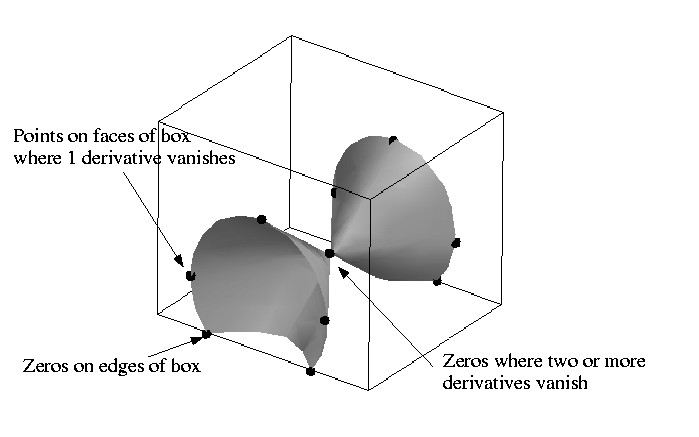# Resolving Faces• Subdivide each face into four sub-faces
• Find solutions on the edges of the faces
• Check for simple cases where no derivatives vanish
• Subdivide more complicated faces. Stop at pre-set depth
• Link together all solutions found.

# Problem Faces

For some situations it can be hard to resolve the faces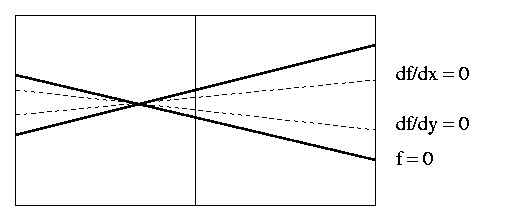In both cases two derivatives vanish in each box.

However, only the left hand box should be marked as containing a node.

This situation cannot be resolved by finer subdivision

# Finding Singularities

Used to find two different types of solution:

• those where two derivatives vanish (north pole on a sphere).
• real singularities where all three derivatives vanish.

Recursive subdivision of the boxes is used to find solutions where two or more derivatives vanish.

Often many false singularities found.

# Constructing the skeleton

Once the solutions have been found a skeleton of the surface can be produces.Information gathered in the previous steps used to find how solutions link together.

# Constructing the polygonization

• First find chains of solutions on the edges and faces of the box
• Then grow the polygons inwards along the edges of the skeleton
Not 100% reliable as there can be many singularities inside a box.

# History

The program was first written in 1992 and used the Geomview visualisation program.

In 1999 it was adapted to run as server producing VRML models, in a client-server system.

In 2000/2001 it was modified to produce JVX models and a user interface was constructed using the Javaview package.

1. Runs on any platform.
Does not require specialist software/hardware.
2. 3D models can give a better feel for the surface.
Allows particular regions to be examined closely.
3. Interactive system allows parametrised families to be examined.

1. Initial load time can be long.
2. Not as visually appealing pictures as those produced by a ray tracer.
3. Does not produce 100% accurate models.
4. Java security restrictions mean that the web-page, applet and server must be on the same host.

# Extensions

1. Non polynomial equations
2. Stand-alone system on a PC
3. Improve calculation around singular points
4. Add a wider range of types of curves and surface:
• Algebraic curves
• Parametrised curves/surface
• Intersections/clipping (clip existing object by a sphere or any other surface)
• apply mappings to a surface (e.g. projection)